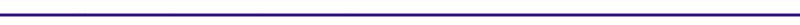Introduction to Engine CyclesSome classic thermodynamic questions that cycle analysis addresses: What is the cylinder pressure and temperature as a function of crank angle? What is the work produced? What is the mean effective pressure? What is the effect of spark timing on the power output ?   The above questions are answered by examining the thermodynamic properties of a piston cylinder system, and the transfer of work and heat into and out of the system. We will use different models to answer the above questions. Each subsequent model increases in realism and complexity from the previous model. The models will all assume a closed system, with no gas exchange. This means that the intake and exhaust strokes of a four cycle engine are not modeled (They are studied in the fluid mechanics section). The strokes to be studied in this section are the compression and expansion strokes. The simplified spark ignition model is the Otto cycle, which assumes all of the energy from the fuel is added to the engine instantaneously at the top dead center. The second model is the Heat Release model, which uses an empirical combustion relation for the combustion gas burn duration as a function of crank angle. The third model is the Heat Release model with heat transfer), which includes heat transfer to the cylinder walls as well as a finite heat release. The simplest compression ignition model is the Diesel cycle, which assumes all of the energy of the fuel is combusted at constant pressure.